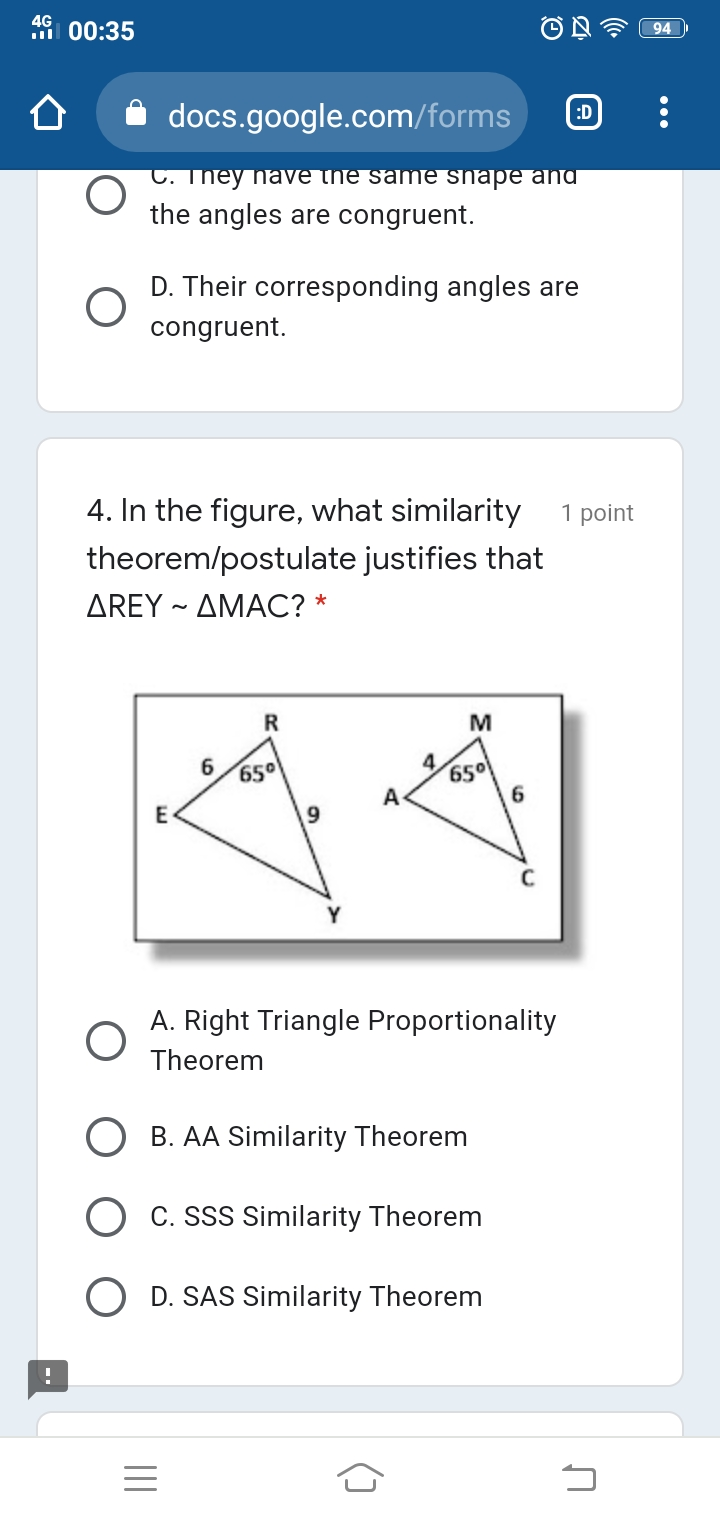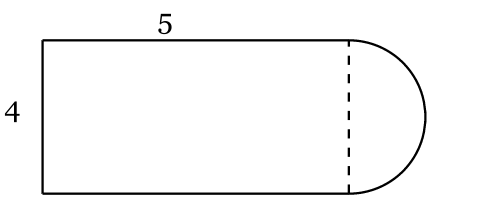# High school geometry questions and answers

Recent questions in High school geometrylifretatox8n 2022-04-07 Answered

2022-04-07

2022-04-06

### In the figure, what similarity theorem/postulate justifies that ΔREY ~ ΔMACOsmarq5ltp 2022-04-06 Answered

### Help with Pythagorean theorem proof for Hilbert SpacesFor given functions f and g in a Hilbert space ${L}^{2}\left(a,b\right)$, prove if the Pythagorean theorem is true for f and g, then it is also true for cf and cg, where c is a constant. What would $$ be?Assume the Pythagorean Theorem holds for functions f and g in a Hilbert space ${L}^{2}\left(a,b\right)$. Then $||f|{|}^{2}+||g|{|}^{2}=||f+g|{|}^{2}$.I have the beginning of the proof above however I am having trouble continuing.poklanima5lqp3 2022-04-06 Answered

### Let us consider a linear programming problem shown below:$\begin{array}{r}\underset{x}{min}f\left(x\right)-c\end{array}$where, $f\left(x\right)$ is a linear function of $x$. Now, can be following maximization problem be considered as an equivalent to the above minimization problem?$\begin{array}{r}\underset{x}{max}\left(\frac{1}{f\left(x\right)-c}\right)\end{array}$terrasson81sgt 2022-04-06 Answered

### Consider the example of maximizing ${x}^{2}yz$ under the constraint that ${x}^{2}+{y}^{2}+{z}^{2}=5$.One way to do this is to use lagrange multipliers, solving the system of equations$2xyz=2x\lambda$${x}^{2}z=2y\lambda$${x}^{2}y=2z\lambda$${x}^{2}+{y}^{2}+{z}^{2}=5$However, couldn't you just substitute ${x}^{2}=5-{y}^{2}-{z}^{2}$ into the expression you want to maximize to get: $yz\left(-{y}^{2}-{z}^{2}+5\right)$and then just maximize that by setting the $y$ and $z$ partial derivatives equal to zero?Then you just have to solve the arguably simpler system of equations:$3{y}^{2}z+{z}^{3}=5z$${y}^{3}+3y{z}^{2}=5y$where the $z$s and $y$s cancel out nicely on both sides.Why is maximize by lagrange multipliers necessary when you can always substitute and maximize the resulting function?When should you choose one over the other?Hailee Stout 2022-04-06 Answered

### It is not possible for a part of any of three conic sections to be an arc of a circle. It is asked to prove this theorem without using any notation or any modern form of symbolism whatsoever (algebra). But, to me, this seems to be quite a difficult task. How can this be done?Karissa Sosa 2022-04-06 Answered

### Let $w,a\in {R}^{n}$, and $B\in {R}_{++}^{n×n}$ (the set of $n×n$ positive definite matrices).We know that the following function (which is a specific form of the Rayleigh quotient) has a unique maximum, and a closed-form solution in $w$:$f\left(w\right)=\frac{{w}^{T}a{a}^{T}w}{{w}^{T}Bw}$It's maximum is achieved at ${w}^{\ast }={B}^{-1}a$ and its value is $f\left({w}^{\ast }\right)={a}^{T}{B}^{-1}a$. (by using the generalized eigenvalue decomposition)Now here is my question:If I have $w,{a}_{1},{a}_{2}\in {R}^{n}$, and ${B}_{1},{B}_{2}\in {R}_{++}^{n×n}$, and the following function:$g\left(w\right)=\frac{{w}^{T}{a}_{1}{a}_{1}^{T}w}{{w}^{T}{B}_{1}w}+\frac{{w}^{T}{a}_{2}{a}_{2}^{T}w}{{w}^{T}{B}_{2}w}$Then, what can we say about the maximum of $g\left(w\right)$, can we still solve for $w$ in closed-form?kromo8hdcd 2022-04-06 Answered

### Suppose I'm given two points: $\left({x}_{1},{y}_{1}\right)$ and $\left({x}_{2},{y}_{2}\right)$ (which are real numbers) lying on the circumference of a circle with radius r and centred at the origin, how do I find the arc length between those two points (the arc with shorter length)?Eve Dunn 2022-04-06 Answered

### I am trying to figure out what the best method is to go about finding this locus.$\mathrm{arg}\frac{z-a}{z-b}=\theta$I am aware that it must be part of an arc of a circle that passes through the points $a$ and $b$. The argument means that the vector $\left(z-a\right)$ leads the vector $\left(z-b\right)$ by $\theta$ and so by the argument must lie on an arc of a circle due to the converse of 'angles in the same segment theorem'.My question is, how do I know what the circle will look like, ie. the centre of the circle and the radius. If I don't need to know this, how will I know what the circle looks like.Finally, if I changed the locus to$\mathrm{arg}\frac{z-a}{z-b}=-\theta$Would this just be the other part of the same circle as previously or a different circle?Perhaps you could help by showing my how it would work with the question$\mathrm{arg}\frac{z-2j}{z+3}=\pi /3$Flakqfqbq 2022-04-06 Answered

### Given matrix-valued function $A:{\mathbb{R}}^{d}\to {\mathbb{R}}^{n×n}$ and (tall) matrix $B\in {\mathbb{R}}^{n×m}$, where $n>m$, let matrix-valued function $C:{\mathbb{R}}^{d}\to {\mathbb{R}}^{m×m}$ be defined by$C\left(x\right):={B}^{T}A\left(x\right)B$I would like to maximize the determinant of $C\left(x\right)$ without directly considering $C\left(x\right)$. If I maximize the determinant of $A\left(x\right)$, does that maximize the determinant of $C\left(x\right)$ as well?If it were $m=n$, then we would have:$det\left(C\right)=det\left(B{\right)}^{2}det\left(A\right)$Hence, maximising $det\left(A\right)$ would maximize as a consequence $det\left(C\right)$. Does it hold something similar in the case with $n>m$? Or is it possible to define some lower bound for $det\left(C\right)$? And if so, how to prove it?If in the previous general case it doesn't hold, maybe it could help that, in my specific case $C$ and $A$ are two positive definite matrices and $B$ is a sparse matrix of zeros and ones. $A$ is also block diagonal. The only way I came up with is using Cauchy-Binet for the determinant of the product of rectangular matrices but I remained stuck with a summation involving the principal minors of the Cholesky factorization of $A$ times minors in $B$.kazue72949lard 2022-04-06 Answered

### I need help with the following optimization problem$max\phantom{\rule{thickmathspace}{0ex}}\alpha \mathrm{ln}\left(x\left(1-{y}^{2}\right)\right)+\left(1-\alpha \right)\mathrm{ln}\left(z\right)$where the maximization is with respect to $x,y,z$, subject to$\begin{array}{rl}\alpha x+\left(1-\alpha \right)z& ={C}_{1}\\ \alpha y\sqrt{x\left(x+\gamma \right)}-\alpha x& ={C}_{2}\end{array}$where $0\le \alpha \le 1$, $\gamma >0$, and $x,z\ge 0$, and $|y|\le 1$.Generally, one can substitute the constraints in the objective function and maximize with respect to one parameter. The problem is that in this way things become algebraically complicated, and I believe that there is a simple solution.briannalofton27 2022-04-01

2022-03-29

###Jewel Dacumos 2022-03-29 Answered

### Determine whether each statement is true or false. If it is false, provide a counterexample. 1.If a quadrilateral is equilateral, then it is equiangular. 2. If a quadrilateral is equiangular, then it is equilateral. 3. Every isosceles triangle has at least one line of symmetry. 4. Every equilateral triangle has exactly three lines of symmetry. 5. If two angles are not congruent, then the two angles are not right.Deegan Chase 2022-03-28 Answered